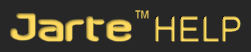Inserting EquationsContents   |    Search   |    Support   |    Jarte Home

 Main menu: Insert > Insert Equation Insert > Insert Equation Edit > Insert Equation

Insert Equation displays an equation editor used to create mathematical equations in your documents. Jarte uses the MathType equation editor to insert equations. The MathType equation editor must already be installed on your computer. If you use an equation editor program other than MathType, you can direct Jarte to use the alternative program by modifying the equation editor advanced setting.

You can modify an existing equation in a document by double clicking the equation.Carolina Road Software